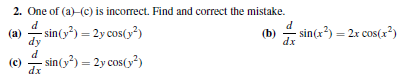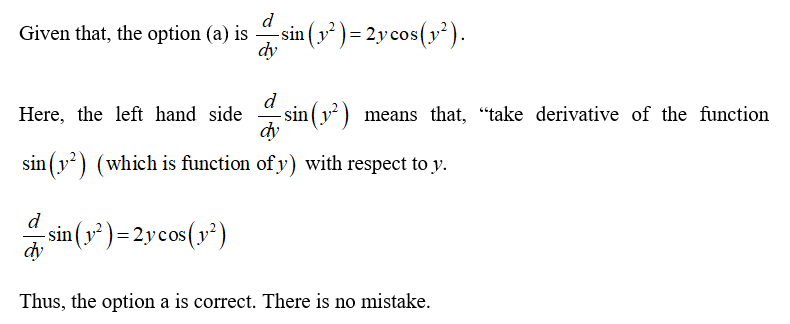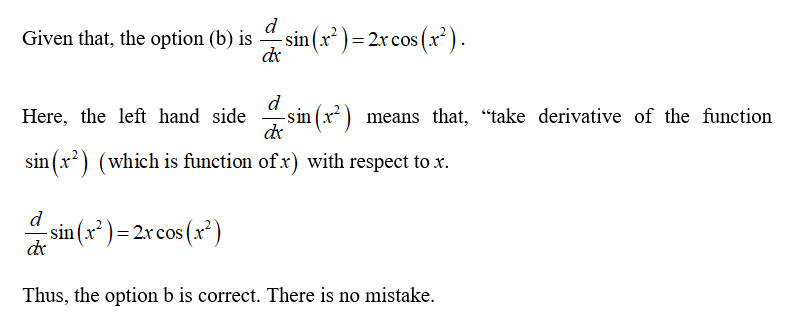# 2. One of (a)-(c) is incorrect. Find and correct the mistake.(a)- sin(y³) = 2y cos(y?)(b)dxsin(x²) = 2x cos(x?)dy(c)sin(y?) = 2y cos(,?)dx

Question
1 viewshelp_outlineImage Transcriptionclose2. One of (a)-(c) is incorrect. Find and correct the mistake. (a) - sin(y³) = 2y cos(y?) (b) dx sin(x²) = 2x cos(x?) dy (c) sin(y?) = 2y cos(,?) dx fullscreen
check_circle

Step 1Step 2...

### Want to see the full answer?

See Solution

#### Want to see this answer and more?

Solutions are written by subject experts who are available 24/7. Questions are typically answered within 1 hour.*

See Solution
*Response times may vary by subject and question.
Tagged in